﻿ 基于界面层模型的三维界面裂纹分析 Layer-Model-Based Three-Dimensional Interface Crack Analysis

International Journal of Mechanics Research
Vol.07 No.02(2018), Article ID:25451,8 pages
10.12677/IJM.2018.72005

Layer-Model-Based Three-Dimensional Interface Crack Analysis

Shuo Wang, Zheng Zhang

Institute of Solid Mechanics, Beihang University, Beijing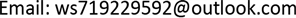Received: May 28th, 2018; accepted: Jun. 8th, 2018; published: Jun. 15th, 2018ABSTRACT

In the field of engineering, interface problems exist extensively, and the special nature of the interface leads to the destruction of bonded materials that often begins at this location. Therefore, in order to ensure the safety of the entire material, the interface crack must be studied. Different from the homogeneous material cracks, the interface cracks show some special properties, such as the oscillation singularity of the field stress at the crack tip, and the dimension of the stress intensity factor. Numerous scholars also proposed a new theory to improve the existing deficiencies. Considering the special properties of interfacial cracks, according to the interface layer theory, the interface of bonded materials is regarded as a functionally graded material (FGM) layer with thickness, and a theoretical system of fracture mechanics for the elliptic cracks in the interfacial layer is established for analysis, which provides a theoretical method for the study of three-dimensional interface crack failure mechanism.

Keywords:Interface Cracks, Functionally Graded Materials, Three-Dimensional Fracture Theory1. 引言

2. 界面层理论

Williams  利用均质材料裂纹的理论解，对界面裂纹尖端场进行研究，由于界面两侧材料性质不同，发现直接应用断裂力学的理论模型存在振荡奇异性等不合理的情况，即在理论上裂纹尖端上下表面互相嵌入，如图1所示，这显然不符合物理常识。

3. 三维界面裂纹模型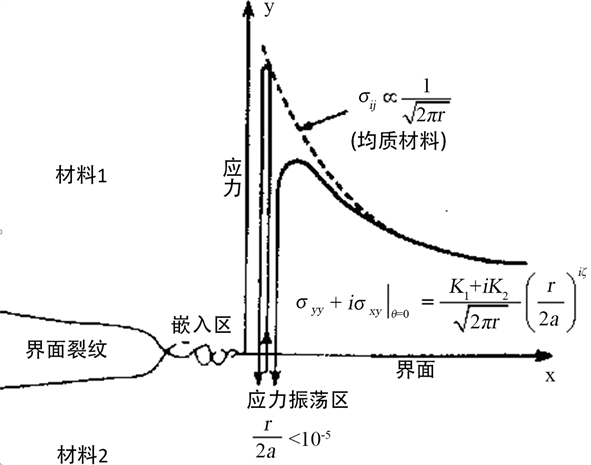Figure 1. Oscillation singularity of interfacial crack stress field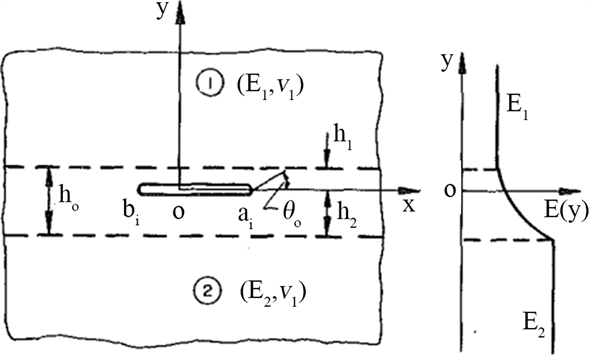Figure 2. Diagram of interface layer model 

$\frac{{x}^{2}}{{a}^{2}}+\frac{{z}^{2}}{{b}^{2}}\le 1,\text{\hspace{0.17em}}\text{\hspace{0.17em}}y=0$ (1)

$x=a\mathrm{cos}\varphi ,\text{ }z=b\mathrm{sin}\varphi$ (2)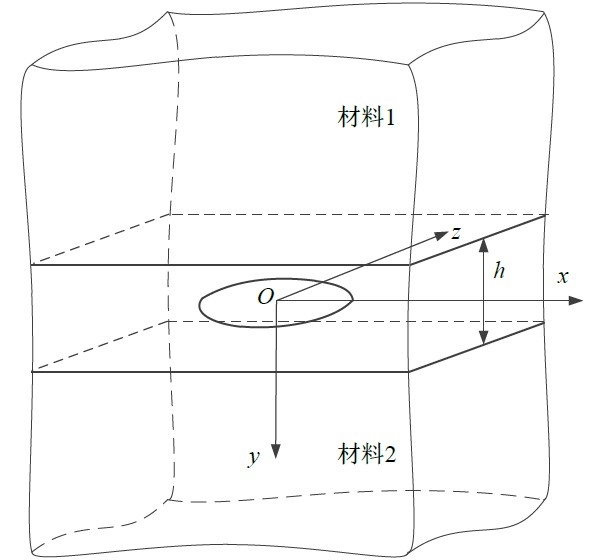Figure 3. Diagram of three-dimensional interface layer model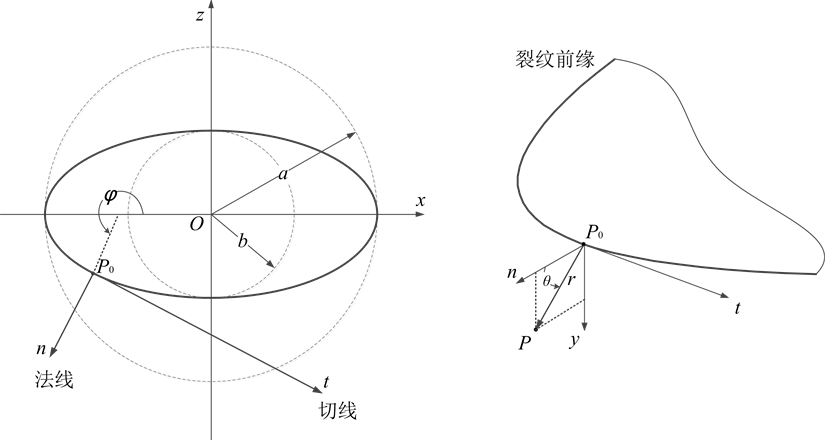Figure 4. Coordinate system in three-dimensional model

$\left\{\begin{array}{l}x=\frac{a\mathrm{cos}\phi }{\sqrt{1-{e}^{2}{\mathrm{sin}}^{2}\phi }}+r\mathrm{cos}\theta \mathrm{cos}\phi \\ y=r\mathrm{sin}\theta \\ z=\frac{{b}^{2}\mathrm{sin}\phi }{a\sqrt{1-{e}^{2}{\mathrm{sin}}^{2}\phi }}+r\mathrm{cos}\theta \mathrm{sin}\phi \end{array}$ ，其中 ${e}^{2}=\frac{{a}^{2}-{b}^{2}}{{a}^{2}}$ 为椭圆离心率。 (3)

4. 求解三维界面裂纹问题

4.1. 三维界面裂纹的弹性理论方程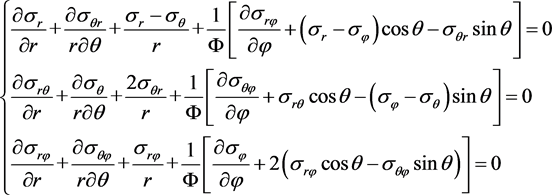(4)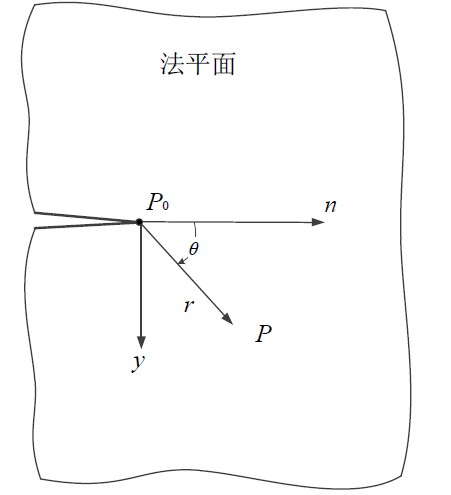Figure 5. Local coordinate system in the front of crack

$\frac{1}{\Phi }={\left[\frac{{b}^{2}}{a\Psi }+r\mathrm{cos}\theta \right]}^{-1},\text{ }\Psi ={\left(1-{e}^{2}{\mathrm{sin}}^{2}\phi \right)}^{3/2}$

$\left\{\begin{array}{l}{\epsilon }_{r}=\frac{\partial {u}_{r}}{\partial r},\text{ }{\epsilon }_{r\theta }=\frac{\partial {u}_{r}}{r\partial \theta }+\frac{\partial {u}_{\theta }}{\partial r}-\frac{{u}_{\theta }}{r}\\ {\epsilon }_{\theta }=\frac{\partial {u}_{\theta }}{r\partial \theta }+\frac{{u}_{r}}{r},\text{ }{\epsilon }_{r\phi }=\frac{\partial {u}_{r}}{\Phi \partial \phi }+\frac{\partial {u}_{\phi }}{\partial r}-\frac{{u}_{\phi }}{\Phi }\mathrm{cos}\theta \\ {\epsilon }_{\phi }=\frac{1}{\Phi }\left(\frac{\partial {u}_{\phi }}{\partial \phi }+{u}_{r}\mathrm{cos}\theta -{u}_{\theta }\mathrm{sin}\theta \right),\text{ }{\epsilon }_{\theta \phi }=\frac{\partial {u}_{\theta }}{\Phi \partial \phi }+\frac{\partial {u}_{\phi }}{r\partial \theta }+\frac{{u}_{\phi }}{\Phi }\mathrm{sin}\theta \end{array}$ (5)

$\begin{array}{l}{\sigma }_{r}=\lambda \Theta +2\mu {\epsilon }_{r},\text{ }{\sigma }_{r\theta }=\mu {\epsilon }_{r\theta }\\ {\sigma }_{\theta }=\lambda \Theta +2\mu {\epsilon }_{\theta },\text{ }{\sigma }_{\theta \phi }=\mu {\epsilon }_{\theta \phi }\\ {\sigma }_{\phi }=\lambda \Theta +2\mu {\epsilon }_{\phi },\text{ }{\sigma }_{\phi r}=\mu {\epsilon }_{\phi r}\end{array}$ (6)

$\mu \left(y\right)=\frac{E\left(y\right)}{2\left(1+\nu \right)},\text{ }\lambda =\frac{\nu }{1-2\nu }\frac{E\left(y\right)}{1+\nu }$ (7)

${\sigma }_{\theta }=0,\text{ }{\sigma }_{\theta r}=0,\text{ }{\sigma }_{\theta \phi }=0\text{ }当\theta =±\text{π}$ (8)

4.2. 平衡方程的简化

$\mu \left(r,\theta \right)=kr\mathrm{sin}\theta +{\mu \left(r,\theta \right)|}_{\theta =0}或\mu =ky+{\mu }_{0}$ (9)

4.2.1. 远场应力的简化计算

$\begin{array}{l}{u}_{x}={\overline{u}}_{x}\left(x,z\right)-y\frac{\partial w\left(x,z\right)}{\partial x}\\ {u}_{y}=y\cdot w\left(x,z\right)\\ {u}_{z}={\overline{u}}_{z}\left(x,z\right)-y\frac{\partial w\left(x,z\right)}{\partial z}\end{array}$ (10)

$\begin{array}{l}\frac{\partial {u}_{x}}{\partial y}=-\frac{\partial w\left(x,\text{ }\text{​}z\right)}{\partial x}\\ \frac{\partial {u}_{y}}{\partial y}=w\left(x,\text{​}\text{ }z\right)\\ \frac{\partial {u}_{z}}{\partial y}=-\frac{\partial w\left(x,\text{​}\text{ }z\right)}{\partial z}\end{array}$ (三者均与y坐标无关) (11)

$\left\{\begin{array}{l}\frac{2\nu \left(ky+{\mu }_{0}\right)}{1-2\nu }\left(\frac{{\partial }^{2}{\overline{u}}_{x}}{\partial {x}^{2}}-y\frac{{\partial }^{3}w}{\partial {x}^{3}}+\frac{{\partial }^{2}{\overline{u}}_{z}}{\partial x\partial z}-y\frac{{\partial }^{3}w}{\partial x\partial {z}^{2}}+\frac{\partial w}{\partial x}\right)+2\left(ky+{\mu }_{0}\right)\left(\frac{{\partial }^{2}{\overline{u}}_{x}}{\partial {x}^{2}}-y\frac{{\partial }^{3}w}{\partial {x}^{3}}\right)\\ +\left(ky+{\mu }_{0}\right)\left(\frac{{\partial }^{2}{\overline{u}}_{x}}{\partial {z}^{2}}+\frac{{\partial }^{2}{\overline{u}}_{z}}{\partial x\partial z}-2y\frac{{\partial }^{3}w}{\partial x\partial {z}^{2}}\right)+k\left(y\frac{\partial w}{\partial x}-\frac{\partial w}{\partial x}\right)+\left(ky+{\mu }_{0}\right)\frac{\partial w}{\partial x}=0\\ \left(ky+{\mu }_{0}\right)\left(\frac{{\partial }^{2}{\overline{u}}_{x}}{\partial x\partial z}+\frac{{\partial }^{2}{\overline{u}}_{z}}{\partial {x}^{2}}-2y\frac{{\partial }^{3}w}{\partial {x}^{2}\partial z}\right)+\frac{2\nu \left(ky+{\mu }_{0}\right)}{1-2\nu }\left(\frac{{\partial }^{2}{\overline{u}}_{x}}{\partial x\partial z}-y\frac{{\partial }^{3}w}{\partial {x}^{2}\partial z}+\frac{{\partial }^{2}{\overline{u}}_{z}}{\partial {z}^{2}}-y\frac{{\partial }^{3}w}{\partial {z}^{3}}+\frac{\partial w}{\partial z}\right)\\ \text{ }+2\left(ky+{\mu }_{0}\right)\left(\frac{{\partial }^{2}{\overline{u}}_{z}}{\partial {z}^{2}}-y\frac{{\partial }^{3}w}{\partial {z}^{3}}\right)+\left(ky+{\mu }_{0}\right)\frac{\partial w}{\partial z}+k\left(-\frac{\partial w}{\partial z}+y\frac{\partial w}{\partial z}\right)=0\\ \left(ky+{\mu }_{0}\right)\left(y\frac{{\partial }^{2}w}{\partial {x}^{2}}-\frac{{\partial }^{2}w}{\partial {x}^{2}}\right)+\left(ky+{\mu }_{0}\right)\left(-\frac{{\partial }^{2}w}{\partial {z}^{2}}+y\frac{{\partial }^{2}w}{\partial {z}^{2}}\right)+\frac{2\nu \left(ky+{\mu }_{0}\right)}{1-2\nu }\left(-\frac{{\partial }^{2}w}{\partial {x}^{2}}-\frac{{\partial }^{2}w}{\partial {z}^{2}}\right)\\ \text{ }+k\left(\frac{\partial {\overline{u}}_{x}}{\partial x}-y\frac{{\partial }^{2}w}{\partial {x}^{2}}+\frac{\partial {\overline{u}}_{z}}{\partial z}-y\frac{{\partial }^{2}w}{\partial {z}^{2}}+w\right)+2kw=0\end{array}$ (12)

4.2.2. 裂尖应力场计算

${\sigma }_{ij}={r}^{n}{f}_{ij}\left({\sigma }_{\infty },a,b,\theta ,\phi \right)$ (13)

$\left\{\begin{array}{l}n{f}_{rr}+\frac{\partial {f}_{\theta r}}{\partial \theta }+{f}_{rr}-{f}_{\theta \theta }+\frac{r}{\Phi }\left[\frac{\partial {f}_{r\phi }}{\partial \phi }+\left({f}_{rr}-{f}_{\phi \phi }\right)\mathrm{cos}\theta -{f}_{\theta r}\mathrm{sin}\theta \right]=0\\ n{f}_{r\theta }+\frac{\partial {f}_{\theta \theta }}{\partial \theta }+2{f}_{\theta r}+\frac{r}{\Phi }\left[\frac{\partial {f}_{\theta \phi }}{\partial \phi }+{f}_{r\theta }\mathrm{cos}\theta -\left({f}_{\phi \phi }-{f}_{\theta \theta }\right)\mathrm{sin}\theta \right]=0\\ n{f}_{r\phi }+\frac{\partial {f}_{\theta \phi }}{\partial \theta }+{f}_{r\phi }+\frac{r}{\Phi }\left[\frac{\partial {f}_{\phi \phi }}{\partial \phi }+2\left({f}_{r\phi }\mathrm{cos}\theta -{f}_{\theta \phi }\mathrm{sin}\theta \right)\right]=0\end{array}$ (14)

${\sigma }_{ij}=\frac{1}{\sqrt{r}}{f}_{ij}\left({\sigma }_{\infty },a,b,\theta ,\phi \right)$ (15)

5. 总结

Layer-Model-Based Three-Dimensional Interface Crack Analysis[J]. 力学研究, 2018, 07(02): 36-43. https://doi.org/10.12677/IJM.2018.72005

1. 1. 于慧子. 二维界面端应力奇异性及界面裂纹分析[D]: [硕士学位论文]. 沈阳: 东北大学, 2014.

2. 2. 嵇醒. 断裂力学判据的评述[J]. 力学学报, 2016, 48(4): 741-753.

3. 3. 许金泉. 界面力学[M]. 北京: 科学出版社, 2006.

4. 4. Gupta, A. and Talha, M. (2015) Recent Development in Modeling and Analysis of Functionally Graded Materials and Structures. Progress in Aerospace Sciences, 79, 1-14. https://doi.org/10.1016/j.paerosci.2015.07.001

5. 5. Zhong, Z., Wu, L.Z. and Chen, W.Q. (2010) Progress in the Study on Mechanics Problems of Functionally Graded Materials and Structures. Advances in Mechanics, 40, 528-541.

6. 6. 黄干云, 汪越胜, 余寿文. 功能梯度材料的平面断裂力学分析[J]. 力学学报, 2005, 37(1): 1-8.

7. 7. Ji, X. (2016) SIF-Based Fracture Criterion for Interface Cracks. Acta Mechanica Sinica, 32, 491-496. https://doi.org/10.1007/s10409-015-0551-1

8. 8. 邸克, 杨月诚. 功能梯度界面层模型断裂问题研究进展[J]. 兵器装备工程学报, 2012, 33(10): 116-119.

9. 9. 匡震邦, 马法尚. 裂纹端部场[M]. 西安: 西安交通大学出版社, 2002.